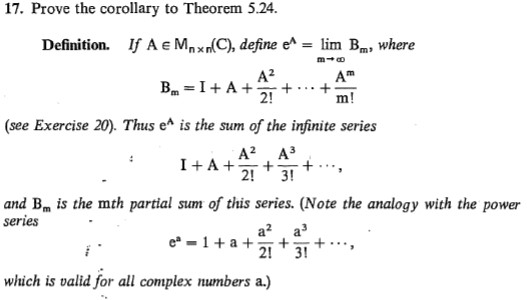# 17. Prove the corollary to Theorem 5.24.If A e Mpxn(C), define e^lim Bm, whereDefinition.A?Bm = I+ A+2!m!(see Exercise 20). Thus e^ is the sum of the infinite seriesА? АЗ-+ ...3!I+A+:2!and Bm is the mth partial sum of this series. (Note the analogy with the powerseriesa? ae* - 1+ a +2!3!which is valid for all complex numbers a.)

Question
1 viewshelp_outlineImage Transcriptionclose17. Prove the corollary to Theorem 5.24. If A e Mpxn(C), define e^ lim Bm, where Definition. A? Bm = I+ A+ 2! m! (see Exercise 20). Thus e^ is the sum of the infinite series А? АЗ -+ ... 3! I+A+: 2! and Bm is the mth partial sum of this series. (Note the analogy with the power series a? a e* - 1+ a + 2! 3! which is valid for all complex numbers a.) fullscreen

### This question hasn't been answered yet.